Inverse Trigonometric Functions

1. If, thenor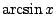is the angle in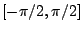whose sine is equal to:.

2. If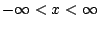, thenoris the angle inwhose tangent is equal to: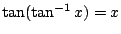.

3. If, then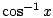or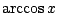is the angle in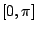whose cosine is equal to:.

Ex 1 Find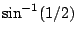and.

Solsinceand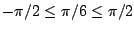, and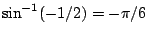sinceand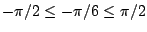.

Ex 2 Find.

Sol Let, so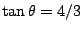. From the right triangle shown below,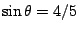, so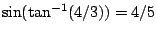.

Pr A Find the following, or explain why they are undefined:

a)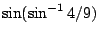and b)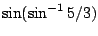.

Pr B Find the following, or explain why they are undefined:

a)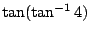and b).

Pr 1 Find a)and b).

Pr 2 Find a)and b).

Pr 3 Find a)and b).

Pr 4 Find.

Pr 5 Find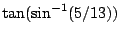.

Pr 6 Find.

Pr 7 Find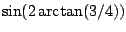.

Pr 8 Find.

Lawrence Marx 2002-07-12